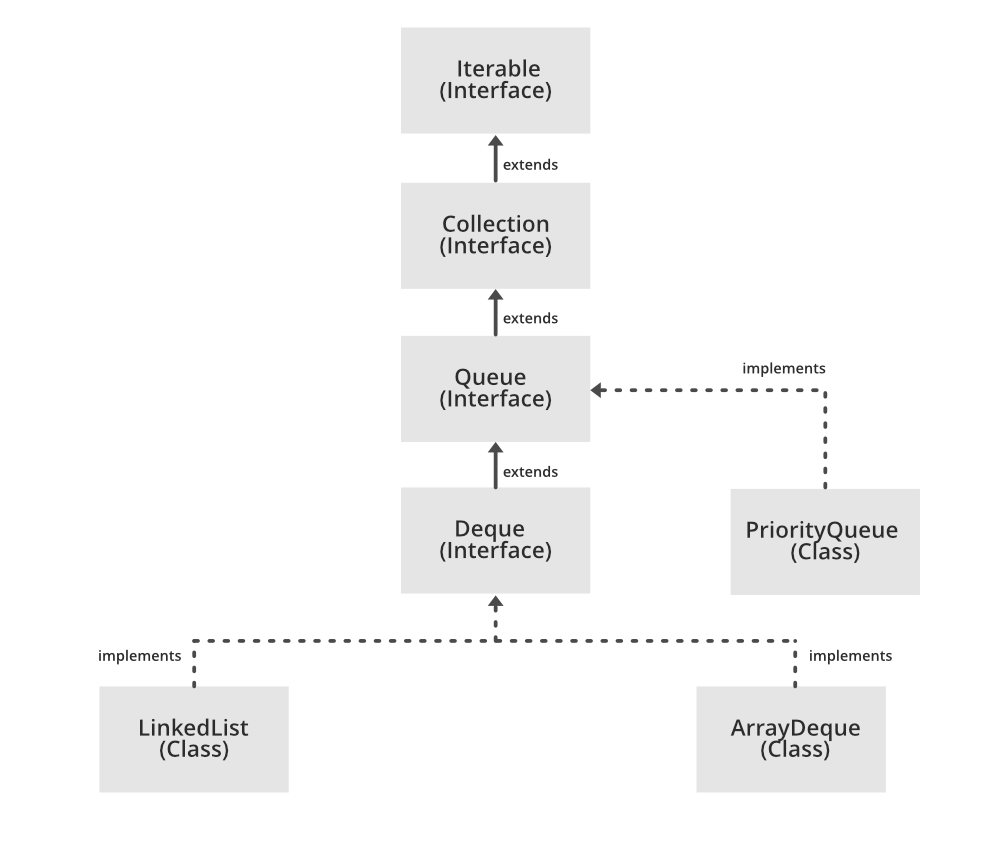# Java Program to Implement PriorityQueue API

• Last Updated : 22 Jul, 2021

A PriorityQueue is a linear data structure in which the elements are ordered according to their natural ordering or by some custom comparator provided at the queue at construction time. In PriorityQueue, the front of the queue points to the least element, and the rear points to the greatest element according to the natural Ordering. For alphabetical PriorityQueue, their ASCII values will be considered for ordering.

Some important characteristics of PriorityQueue are as follows:

• It does not allow the null elements to be inserted.
• It is an unbounded Queue that means its size can be expanded.
• It inherits the classes like Object, Abstract Collection, AbstractQueue.
• It is not thread-safe.
• It cannot be created for non-comparable objects.

Time Complexities of various operations:

• Insertion and deletion are f order O(log(n))
• remove() and contains() method is of order O(n)
• Retrieval operations are the fastest which are of order O(1)

The PriorityQueue class inherits Queue Interface and all of its methods. PriorityQueue API implements serializable, Iterable, Collection, and Queue which can be perceived from the architecture shown below.

`Serializable, Iterable<E>, Collection<E>, Queue<E>`Syntax:

`public class PriorityQueue<E> extends AbstractQueue<E> implements Serializable`

Parameter: E — The type of elements held in this queue.

Methods:

Implementation:

Example

## Java

 `// Java Program to implement Priority Queue API` `// Importing all classes from java.util package``import` `java.util.*;` `// Class``class` `GFG {` `    ``// Main driver method``    ``public` `static` `void` `main(String[] args)``    ``{``        ``// Creating(Declaring) an object of PriorityQueue of``        ``// Integer type i.e Integer elements will be``        ``// inserted in above object``        ``PriorityQueue pq = ``new` `PriorityQueue<>();` `        ``// Adding elements to the object created above``        ``// Custom inputs``        ``pq.add(``89``);``        ``pq.add(``67``);``        ``pq.add(``78``);``        ``pq.add(``12``);``        ``pq.add(``19``);` `        ``// Printing the head of the PriorityQueue``        ``// using peek() method of Queues``        ``System.out.println(``"PriorityQueue Head:"``                           ``+ pq.peek());` `        ``// Display message``        ``System.out.println(``"\nPriorityQueue contents:"``);` `        ``// Defining the iterator to traverse over elements of``        ``// object``        ``Iterator i = pq.iterator();` `        ``// Condition check using hasNext() method which hold``        ``// true till single element is remaining in List``        ``while` `(i.hasNext()) {` `            ``// Printing the elements of object``            ``System.out.print(i.next() + ``" "``);``        ``}` `        ``// Removing random element from above elements added``        ``// from the PriorityQueue``        ``// Custom removal be element equals 12``        ``pq.remove(``12``);` `        ``// Display message``        ``System.out.print(``"\nPriorityQueue contents:"``);` `        ``// Declaring iterator to traverse over object``        ``// elements``        ``Iterator it = pq.iterator();` `        ``// Condition check using hasNext() method which hold``        ``// true till single element is remaining in List``        ``while` `(it.hasNext()) {` `            ``// Printing the elements``            ``System.out.print(it.next() + ``" "``);``        ``}` `        ``// Removing all the elements from the PriorityQueue``        ``// using clear() method``        ``pq.clear();` `        ``// Adding another different set of elements``        ``// to the Queue object``        ``// Custom different inputs``        ``pq.add(``5``);``        ``pq.add(``7``);``        ``pq.add(``2``);``        ``pq.add(``9``);` `        ``// Checking a random element just inserted``        ``// using contains() which returns boolean value``        ``System.out.print(``"The queue has 7 = "``                         ``+ pq.contains(``7``));` `        ``// Display message for content in Priority queue``        ``System.out.print(``"\nPriorityQueue contents:"``);` `        ``// Converting PriorityQueue to array``        ``// using toArray() method``        ``Object[] arr = pq.toArray();` `        ``// Iterating over the array elements``        ``for` `(``int` `j = ``0``; j < arr.length; j++) {` `            ``// Printing all the elements in the array``            ``System.out.print(arr[j] + ``" "``);``        ``}``    ``}``}`

Output

```PriorityQueue Head:12

PriorityQueue contents:
12 19 78 89 67
PriorityQueue contents:19 67 78 89 The queue has 7 = true
PriorityQueue contents:2 7 5 9 ```

My Personal Notes arrow_drop_up